# Combinational Logic Circuits

Tom Kelliher, CS26

Oct. 1, 1996

# Synthesis of Logic Functions

• Given a logic expression, how do we implement it?

• Every Boolean expression can be represented in sum of products form.

• Implementing SOP expressions.

• Limitations.

Convert each of the following to SOP notation and draw a logic circuit, using AND, OR, and inverter gates, implementing it:

1.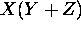.

2. XY + YZ.

3.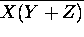.

4.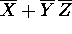.

5. Implement a binary full adder. Here is the truth table: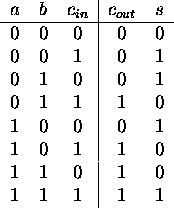Start by writing the SOP equation.

BTW, is this a useful function?

6. Consider a five-input Boolean function that is asserted whenever exactly two of its inputs are asserted. Construct its truth table, its SOP equation(s), and an implementation.

# Minimization

Why bother? Speed, power, real estate.

Minimization Techniques:

1. Quine-McCluskey.

2. Karnaugh Maps.

3. Espresso.

## K Maps

Two-variable example: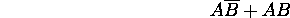1. Minimal cover.

2. The map is a sphere.

3. Don't cares in real circuits.

4. Gray code numbering.

5. Converting the covers to equations.

Try the following:

1. The sum output of a full binary adder.

2. The carry out output of a full binary adder.

3. A circuit which compares two-bit unsigned numbers. There are three outputs: inputs equal, first greater, second greater.

# Synthesis with NAND and NOR Gates

Why do TTL and CMOS designers use these gates?

Why are ECL designers so lucky?

# Exercises

1. Design and implement a 2-1 multiplexer, using a K-map for minimization of the output equation. Here's the truth table: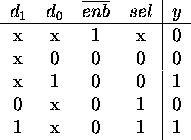A multiplexer works like a switch. One way of drawing them is: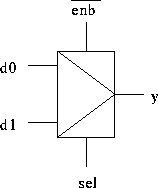2. Design and implement a circuit to take a BCD-encoded digit and drive a seven-segment display (used in watches and calculators). Use a K-map to minimize each of the seven output equations. Here is the labeling for the display: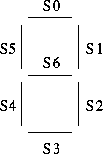Thomas P. Kelliher
Mon Sep 30 09:23:07 EDT 1996
Tom Kelliher